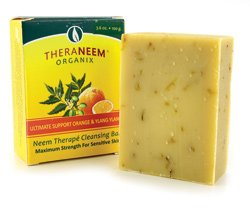# Organix South Ultimate Support W/Orange & Ylang Soap Organix South 4 Oz Bar Soap

• \$24.31
• Save \$6.95

• D

• i

• s

• c

• l

• a

• i

• m

• e

• r

• :

• T

• h

• i

• s

• w

• e

• b

• s

• i

• t

• e

• i

• s

• f

• o

• r

• i

• n

• f

• o

• r

• m

• a

• t

• i

• o

• n

• a

• l

• p

• u

• r

• p

• o

• s

• e

• s

• o

• n

• l

• y

• .

• A

• l

• w

• a

• y

• s

• c

• h

• e

• c

• k

• t

• h

• e

• a

• c

• t

• u

• a

• l

• p

• r

• o

• d

• u

• c

• t

• l

• a

• b

• e

• l

• i

• n

• y

• o

• u

• r

• p

• o

• s

• s

• e

• s

• s

• i

• o

• n

• f

• o

• r

• t

• h

• e

• m

• o

• s

• t

• a

• c

• c

• u

• r

• a

• t

• e

• i

• n

• g

• r

• e

• d

• i

• e

• n

• t

• i

• n

• f

• o

• r

• m

• a

• t

• i

• o

• n

• d

• u

• e

• t

• o

• p

• r

• o

• d

• u

• c

• t

• c

• h

• a

• n

• g

• e

• s

• o

• r

• u

• p

• g

• r

• a

• d

• e

• s

• t

• h

• a

• t

• m

• a

• y

• n

• o

• t

• y

• e

• t

• b

• e

• r

• e

• f

• l

• e

• c

• t

• e

• d

• o

• n

• o

• u

• r

• w

• e

• b

• s

• i

• t

• e

• .

• T

• h

• e

• s

• e

• s

• t

• a

• t

• e

• m

• e

• n

• t

• s

• m

• a

• d

• e

• i

• n

• t

• h

• i

• s

• w

• e

• b

• s

• i

• t

• e

• h

• a

• v

• e

• n

• o

• t

• b

• e

• e

• n

• e

• v

• a

• l

• u

• a

• t

• e

• d

• b

• y

• t

• h

• e

• F

• o

• o

• d

• a

• n

• d

• D

• r

• u

• g

• A

• d

• m

• i

• n

• i

• s

• t

• r

• a

• t

• i

• o

• n

• .

• T

• h

• e

• p

• r

• o

• d

• u

• c

• t

• s

• o

• f

• f

• e

• r

• e

• d

• a

• r

• e

• n

• o

• t

• i

• n

• t

• e

• n

• d

• e

• d

• t

• o

• d

• i

• a

• g

• n

• o

• s

• e

• ,

• t

• r

• e

• a

• t

• Size : 4 oz

Contains 20% certified organic Neem oil! All natural, made with only the finest quality oils. Hand made without synthetic colors, fragrances or preservatives. Neem has been used for thousands of years for its ability to soothe, balance and nourish the skin. This is our most concentrated and beneficial bar, now with fragrant essential oils, for those with skin that is prone to irritation. This mild soap combines the ancient properties of neem with the aesthetics of a delicately hand-crafted soap.

every pages